# Module 1: Properties And Structure Of Matter

What's the matter? You're a little rusty on properties and structures of matter? Don't fear. In this article, we will go through properties of matter, atomic structures and atomic mass and periodicity.This article provides an overview of the Properties and Structure of Matter — the stuff that makes up everything! Use this as part of a study plan to make sure you know all the key concepts associated with this module.

## Properties and structure of matter

This portion of the syllabus provides an introduction to chemistry, being the start of Year 11. Basic differences in states of matter are introduced and considered, and you’ll encounter some new terminology. Make sure you understand all of the new words that are introduced and are able to define them.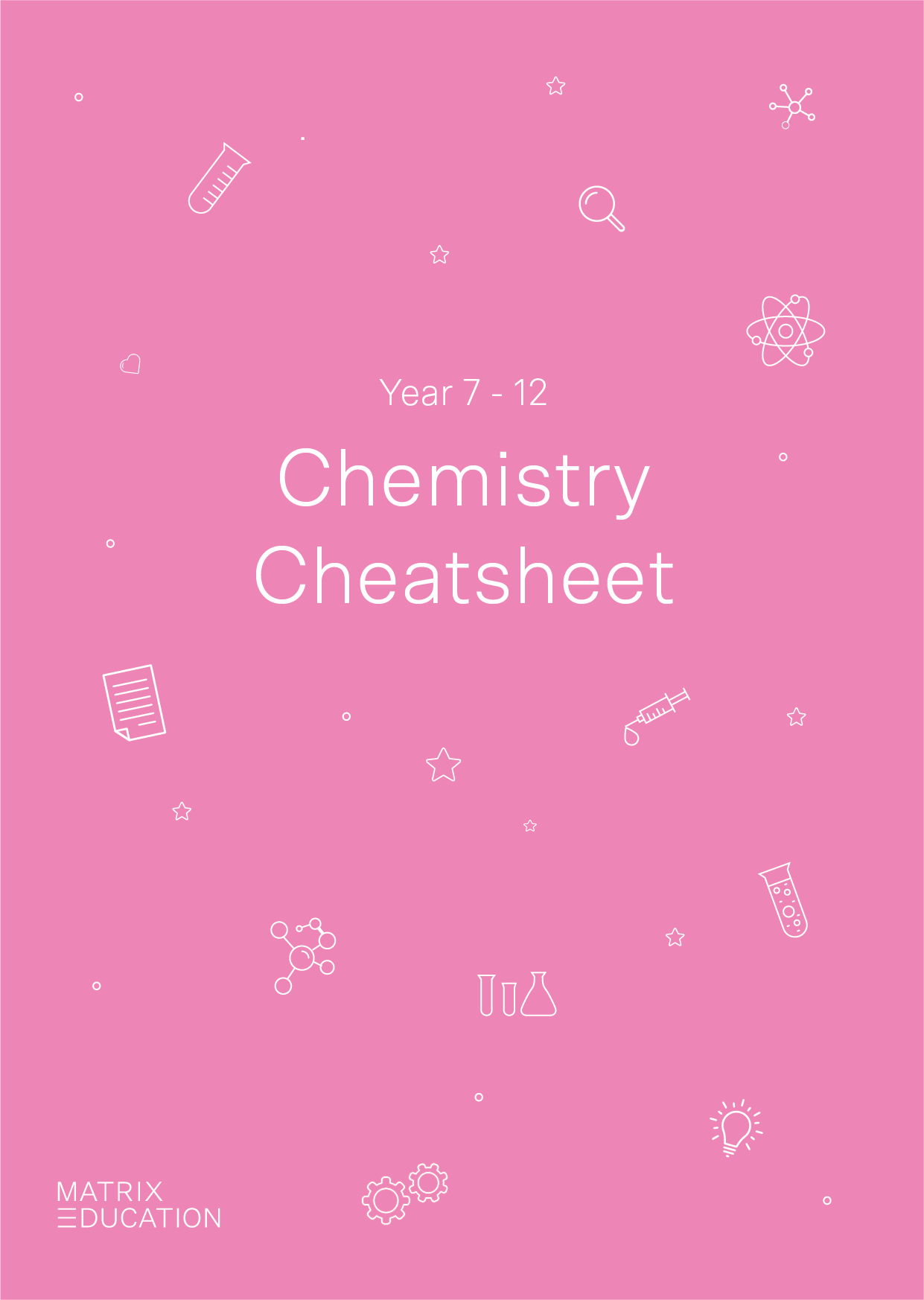A free pocket-sized cheatsheet, so you're always prepared.

### Separating mixtures

A mixture is a composite of two or more substances that are not chemically combined. Importantly, the substances in the mixture keep their individual chemical and physical properties.

There are two types of mixtures:

1. Homogenous: Uniform distribution of component substances (like steel).

2. Heterogenous: Non-uniform distribution of component substances (like mud).

Mixtures can be separated into the individual substances that they are composed of using a number of experimental techniques you should be familiar with.

We’ve illustrated these techniques below with some helpful diagrams.

1. Filtration: Separates solids from liquids using a filter funnel, filter paper, or membrane.2. Sieving: Separates solids of different sizes with a sieve.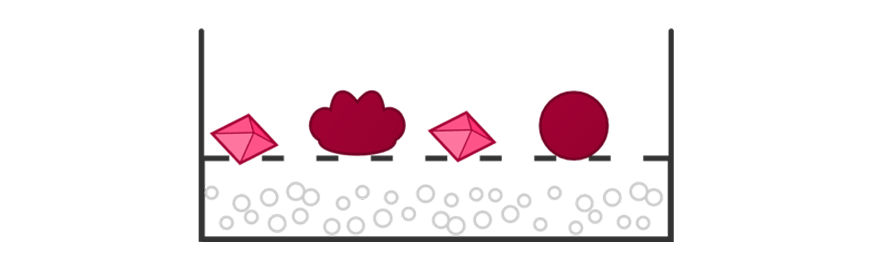3. Distillation: Separates liquids using differences in boiling points with a condenser.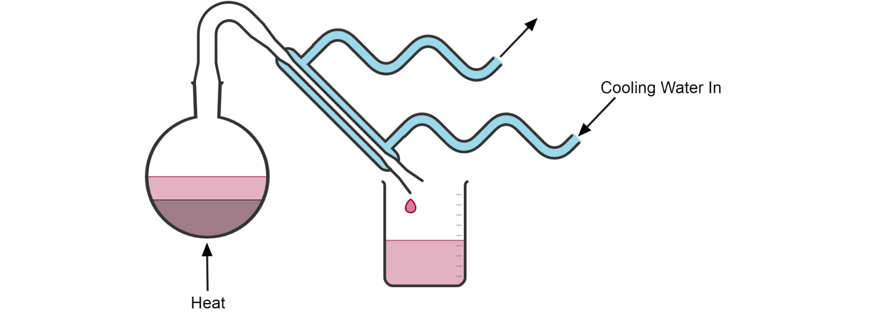4. Evaporation: Separates a solid dissolved in a liquid by boiling off the liquid in an evaporating dish.5. Magnetic Separation: Separates magnetic substances from non-magnetic substances using a permanent magnet or electromagnet.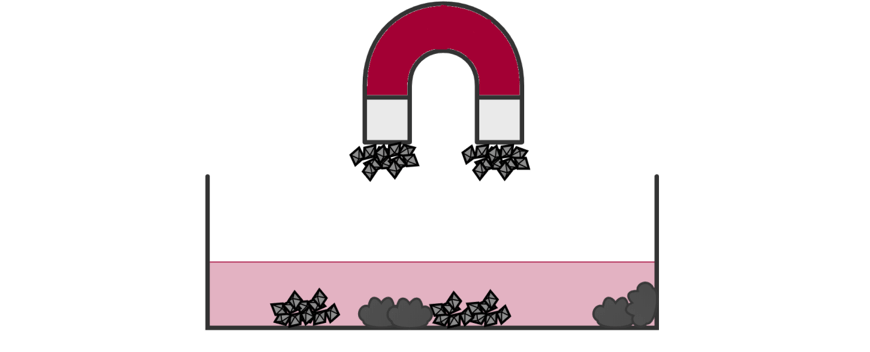To calculate the percentage composition for one substance in a mixture, use the following formula:

$$\text{Percentage composition} = \ \frac{\text{Mass of Substance}}{\text{Total Mass of Mixture}} \ \times \ 100$$

### Classifying elements

Elements are pure substances that are made up of only one type of atom.

It is important that you know how to classify an element (as metal, non-metal or semi-metal) based on the position the element occupies in the periodic table and the physical and chemical properties of the element.

Metals are found on the left-hand side of the periodic table, and generally have high melting points, good electrical and thermal conductivity, are lustrous, malleable and ductile.

Non-metals are found on the right-hand side of the periodic table and generally have low melting points, are poor electrical and thermal conductors, are dull and brittle.

Semi-metals are boron, silicon, germanium, arsenic, antimony, tellurium, and polonium. Semi-metals generally have very high melting points, are semiconductors of electricity, are lustrous and brittle.

Different elements will have very different chemical properties.

You should be aware of the general reactivity of metals, non-metals and semi-metals – but be aware that this is more closely related to the group of the periodic table the element is found in!

The following trends are a good place to start:

• Group 1 metals react with water and oxygen, from cations in salts and are highly reactive.
• Group 2 metals react with water or oxygen with heating, form cations in salts and are somewhat reactive.
• Carbon forms many covalent compounds, some of which have different chemical and physical properties.
• Silicon is a semi-conductor, and also forms many covalent compounds.
• Non-metals vary in the level of reactivity observed, with the exception of the halogens (group 17) which are very reactive, and will gain one electron to achieve a stable valence electron configuration.

You should also be able to identify the state of a given element at room temperature and pressure.

Most elements are solids at room temperature, but mercury and bromine are liquids.

Hydrogen, nitrogen, oxygen, fluorine, chlorine and the group 18 elements are all gases at room temperature and pressure.

## Atomic structure and atomic mass

So we’ve talked a bit about different elements in the above section, and there are heaps of them — 118 in fact!

But what is it that makes an element an element? Why are some different from others?

It all comes down to atomic structure!

So here we’re going to take a deep dive into the composition of atoms and look at what makes them so different from one another.

### The atom

As you are probably aware, atoms are composed of protons, neutrons and electrons.

• Protons carry a positive charge
• Neutrons carry no charge
• Electrons carry a negative charge
• Protons and neutrons happily reside in the nucleus
• Electrons are located in energy levels around the nucleus

The periodic table gives you all the information you need to work out the atomic structure of an element. Have a look at the Key of the IUPAC periodic table if you are unsure about how to read the data provided.

The atomic number is unique to each element, and shows the number of protons present in the nucleus.

The atomic mass of an element is the sum of the number of protons and neutrons. You may notice that most elements do not have integer values of atomic mass in the periodic table. This is because the atomic mass is an average value of a typical sample of the element found on Earth which will contain different isotopes.

An isotope is a variation of an element that contains a different number of neutrons. For example, $$^{16} O$$ contains 8 neutrons, and $$^{18} O$$ contains10 neutrons.

It is possible to calculate the relative atomic mass of an element based upon the amount of each isotope.

To do so, you should use the following formula.

$$\text{Standard Atomic Mass} = \ (\%_A \times M_A ) + (\%_B \times M_B) + (\%_C \times M_C) \text{…}$$

$$\%_A$$ is the percentage abundance of isotope $$A$$ and $$M_A$$ is the mass of isotope  $$A$$ and so on.

A neutral element contains the same number of protons and electrons.

### Electronic Structure of The Atom

There are two ways you need to be able to think about the electronic structure of the atom in the Year 11 Chemistry course, the Bohr model and the Schrödinger model.

1. The Bohr model of the atom states that electrons orbit the nucleus in shells of fixed size and energy.

A schematic of the Bohr model is shown below.2. The Schrödinger model depicts electrons as matter waves, which are described by a wavefunction.

The end result of this is a “zone of probability” in which electrons can be found, which divides the shells of the Bohr model into distinct subshells.

The shapes of the different types of subshells are illustrated below.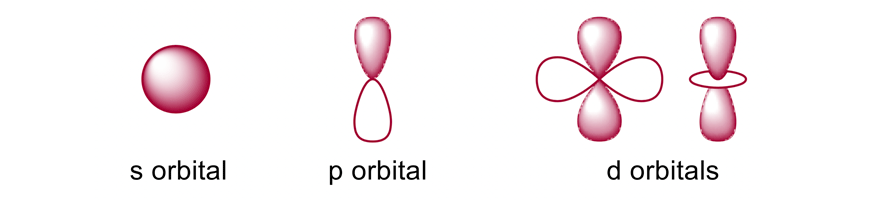The filling order for the Schrödinger model is $$1s^{2} \ 2s^{2} \ 2p^{6} \ 3s^{2} \ 3p^{6} \ 4s^{2} 3d^{10}$$

For example, if we consider elemental chlorine which has 17 electrons, we can depict it using the two diagrams below. The first one shows the Bohr model and the second the Schrödinger model. It’s important that you know how to use both.

• Bohr model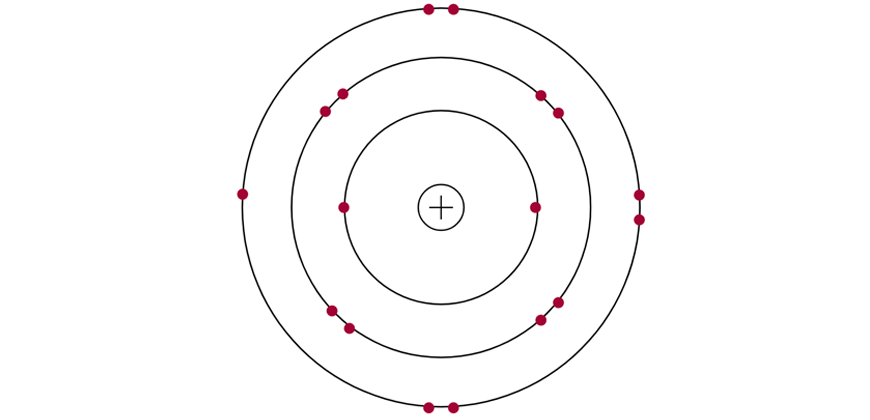$$2, \ 8, \ 8$$

• Schrödinger model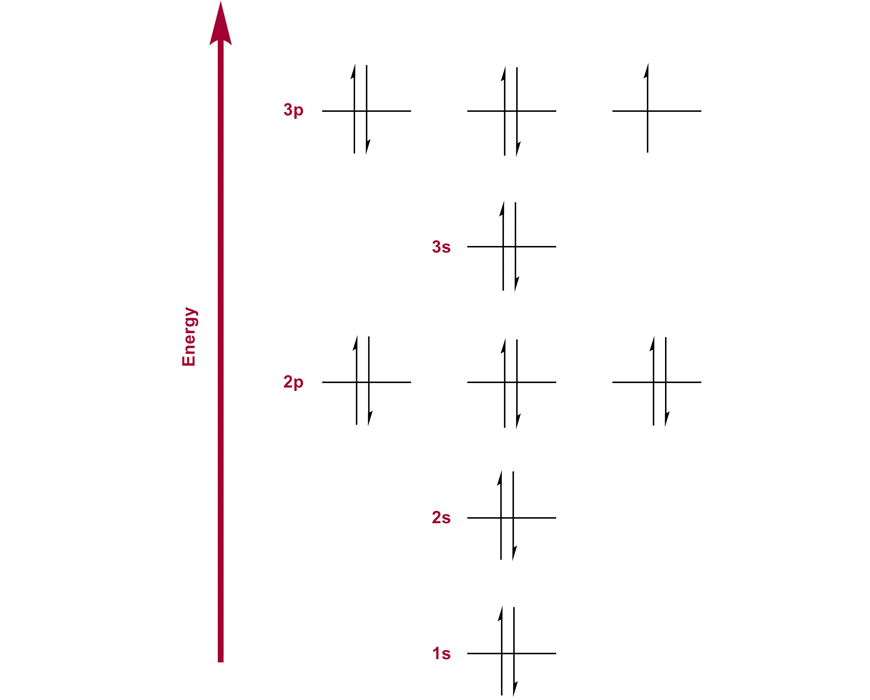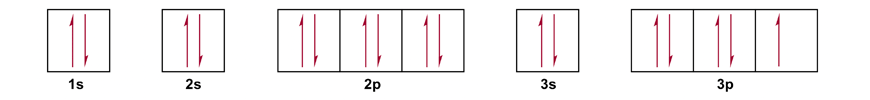$$1s^{2} \ 2s^{2} \ 2p^{6} \ 3s^{2} \ 3p^{5}$$

When constructing an electron spin-orbital diagram using the Schrödinger model, it is important that you adhere to the three following rules:

1. The Aufbau Principle: Orbitals of an atom must be filled from the lowest energy level first.
2. The Pauli Exclusion Principle: No more than two electrons can occupy a single atomic orbital, and paired electrons must be of opposite spin.
3. Hund’s Rule: Every orbital in a subshell must be occupied with one electron before it can be paired with another electron, and the spin of unpaired electrons in singly occupied orbitals must be aligned.

### Flame Tests

A Flame Test is an experiment that can be carried out in order to distinguish samples containing different metallic elements.

In essence, when the element is heated, a specific colour of light is produced.

It is useful to consider the Bohr model of the atom when rationalising the phenomena observed.

1. When an atom in the ground state is heated, the electrons are able to gain a quantised amount of energy, and the atom enters an excited state.
2. The electrons then relax back down to lower energy levels by emitting light of a specific wavelength.
3. This wavelength corresponds to the difference in energy between the shells of the atom.

The diagram below illustrates this process: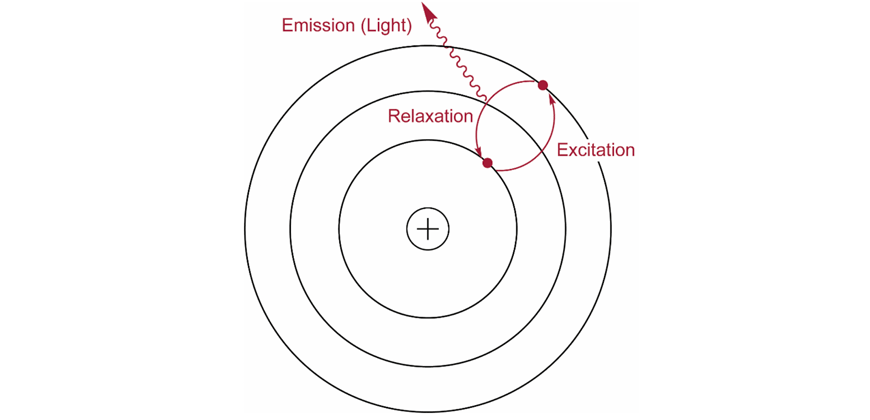### Nuclear chemistry

So far, we’ve spoken about the behaviour of electrons in each element, but now we get to learn more about the behaviour of atomic nuclei:

• When are they stable, and when are they unstable?
• What is this radiation stuff anyway?

First, we need to introduce the concept of a radioisotope – the word is a combination of the words “radioactive” and “isotope”.

So it’s a type of isotope that emits radiation!

For an isotope to be radioactive, it must contain a ratio of protons:neutrons in the nucleus that is unstable.

All elements with an atomic number larger than 82 are radioactive, but some isotopes of regular elements like carbon or oxygen can be radioisotopes if they have too many neutrons.

A perfect example of this is the radioisotope $$^{14}C$$, which has two more neutrons than $$^{12}C$$, and undergoes radioactive decay, emitting radiation.

Below is a chart of some possible combinations of protons and neutrons in the nucleus (nuclei shown in black are stable, and nuclei shown in maroon are unstable). Note that the stable nuclei fall in a narrow range of proton:neutron ratio.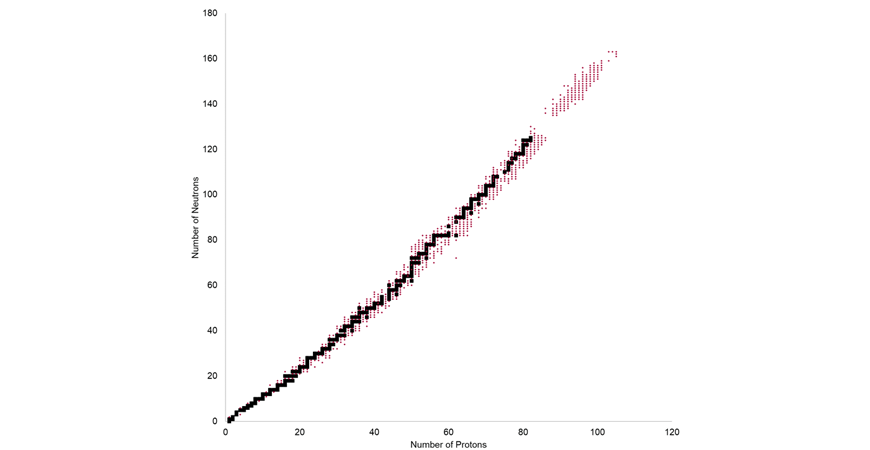Radioactive nuclei will emit radiation when they decay and there are three types of radiation you need to know about: alpha, beta and gamma.

Alpha radiation is composed of a helium nucleus and is represented by the symbol $$_{2}^{4} He$$. It carries a positive charge and is deflected towards the negative plate of an electric field.

Beta radiation is composed of high energy electrons, and is represented by the symbol $$_{-1}^{ \ \ 0} \beta \ \text{or} \ _{-1}^{ \ \ 0}e^{-}$$. It carries a negative charge and is deflected towards the positive plate of an electric field.

Gamma radiation is composed of high energy electromagnetic radiation, and is represented by the symbol $$\gamma$$ – it carries no charge and is not deflected by an electric field.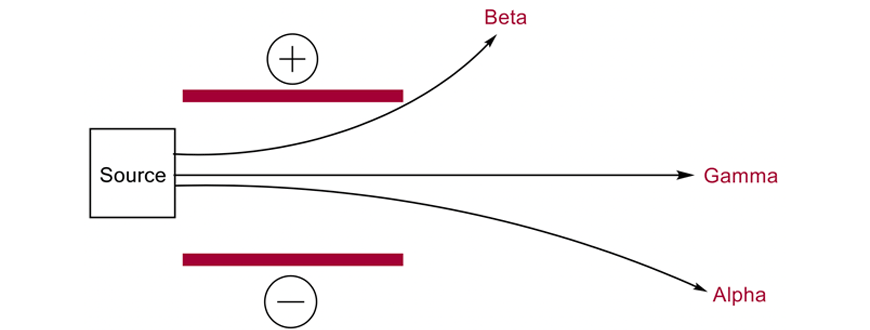We can write equations for nuclear decay in the same way we write equations for chemical reactions. You need to be able to do this for the three different types of radiation. For example, the equation below describes the decay of $$^{14}C$$ to form $$^{14}N$$:

$$_{ \ 6}^{14}C \ \rightarrow \ _{ \ 7}^{14} N \ + \ _{-1}^{ \ \ 0} \beta$$

There are many uses for radioisotopes – radiation doesn’t have to be scary if used in the correct way! For example, iodine-131 can help treat thyroid cancer because it accumulates in the thyroid gland and delivers a localised dose of radiation to kill cancer cells, and americium-241 is used in smoke detectors as an alpha emitter to generate ionised gas.

## Periodicity

Periodicity refers to repeating trends – these repeating trends allowed early chemists to devise a system for classifying elements. The end result is the periodic table produced by Dmitri Mendeleev (this guy).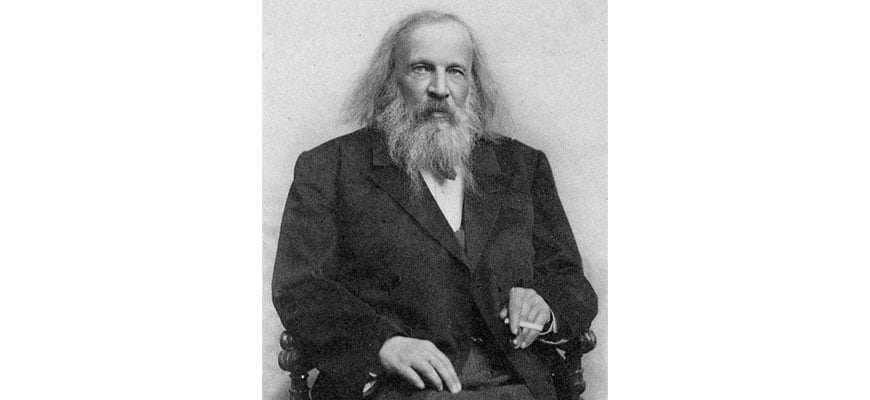### Trends in the periodic table

The elements are arranged in the Periodic Table in such a way that some trends become easy to remember. The trends you need to know are illustrated on the periodic table below.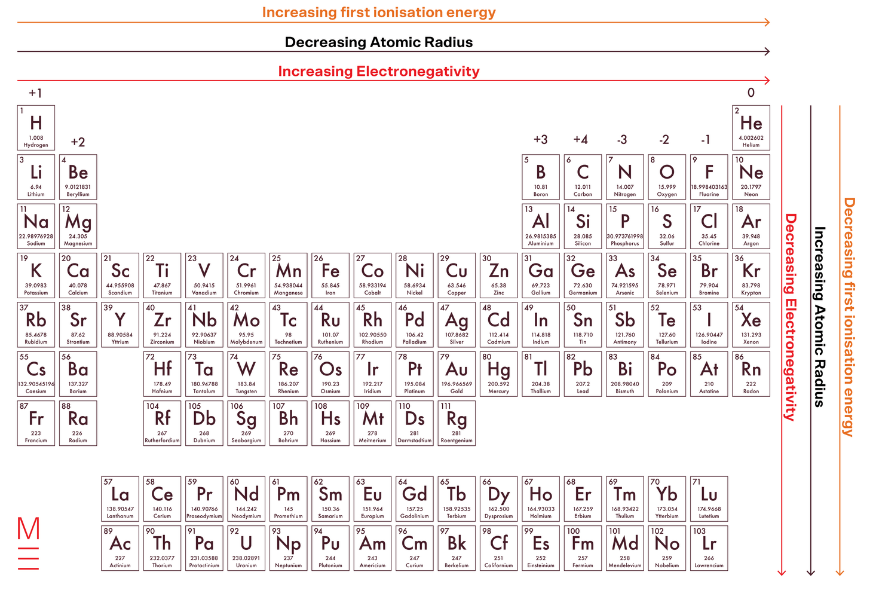The group an element is part of will also determine the valency of the ion the element will form. The charge of the ion formed in each group is shown above the group in the chart above. (You cannot determine the charge of a transition metal ion from the position it occupies in the periodic table.)

### Bonding

In order to understand chemical bonding, we first need to check in on a concept known as electronegativity.

Electronegativity is the ability of an element to attract bonding electrons.

It is crucial that you understand this!

1. A large difference in electronegativity will result in ionic bonding

2. A smaller difference in electronegativity will result in covalent bonding or metallic bonding.

### Naming compounds

When naming inorganic substances, simply list the cation and anion.

When a transition metal is used, list the oxidation state as well.

For example, the name for $$FeCl_3$$ is iron(III) chloride and the name for $$MgSO_4$$is magnesium sulfate.

When naming organic substances, list the elements in order and include prefixes to indicate the number of a given type of atom.

For example, the name of $$PCl_3$$  is phosphorus trichloride and the name of $$N_2O_4$$  is dinitrogen tetroxide.

For Year 11 Chemistry and the HSC, you are expected to know the following prefixes.

 Number Prefix Number Prefix 1 Mono 5 Penta 2 Di 6 Hexa 3 Tri 7 Hepta 4 Tetra 8 Octa

You should also be familiar with writing the formulae of inorganic and organic substances from their names.

### Lewis dot structures

Lewis electron dot structures help us describe covalent bonds within different molecules.

The “dots” in these structures represent valence electrons involved in chemical bonding.

The Lewis dot structures of the 2nd-period elements are shown below.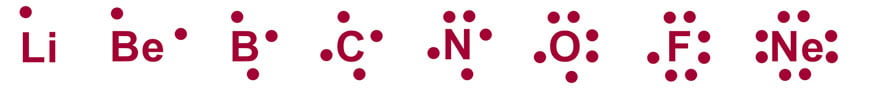When arranging the electrons to form covalent bonds, the aim is to ensure each electron is surrounded by a full valence shell. This is known as the octet rule.

You should be confident in drawing Lewis dot structures for a variety of simple covalent compounds, like those illustrated below.Start by drawing out the Lewis dot structure for each atom, and start sharing electrons.

It’s ok to get it wrong a couple of times and find a solution through trial and error!

### Polarity

Despite the fact that covalent bonds are formed by sharing electrons between different elements, electrons will spend a greater amount of time closer to the more electronegative element.

This creates a permanent dipole in the covalent molecule. One side has a slightly positive charge, and the other a slightly negative charge.

This is often indicated using the Greek lower case delta symbol $$\delta$$.

This property is shown well in a molecule such as $$HCl$$, where electrons hang out around the more electronegative chlorine atom.If the electron distribution in a molecule is asymmetric, we say the molecule is polar.

A molecule with symmetric electron distribution is non-polar.

In a simple diatomic molecule like $$HCl$$, a larger difference in electronegativity will result in a more polar bond.

### VSEPR Theory

We can use our knowledge of drawing Lewis dot structures to predict the shape of a molecule using VSEPR theory.

The principle behind VSEPR is that negatively charged electrons will repel one another, resulting in predictable shapes based on the number of groups and lone pairs attached to the central atom of a molecule.

Let’s have a look at all of the VSEPR shapes you are required to know. We’ve even made some helpful rotating diagrams so you can see them in 3D! (To see the 3D versions, please click the link below the image and it will open it in a new window. Be warned they a large – 2-3Mb)

You are expected to be able to draw the Lewis dot structure for a molecule and identify the shape according to VSEPR theory.

Linear (Two VSEPR groups)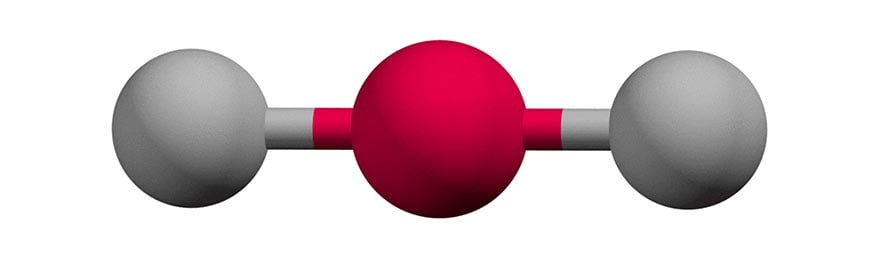Bent / Angular (Three VSEPR groups, one lone pair OR Four VSEPR groups, two lone pairs)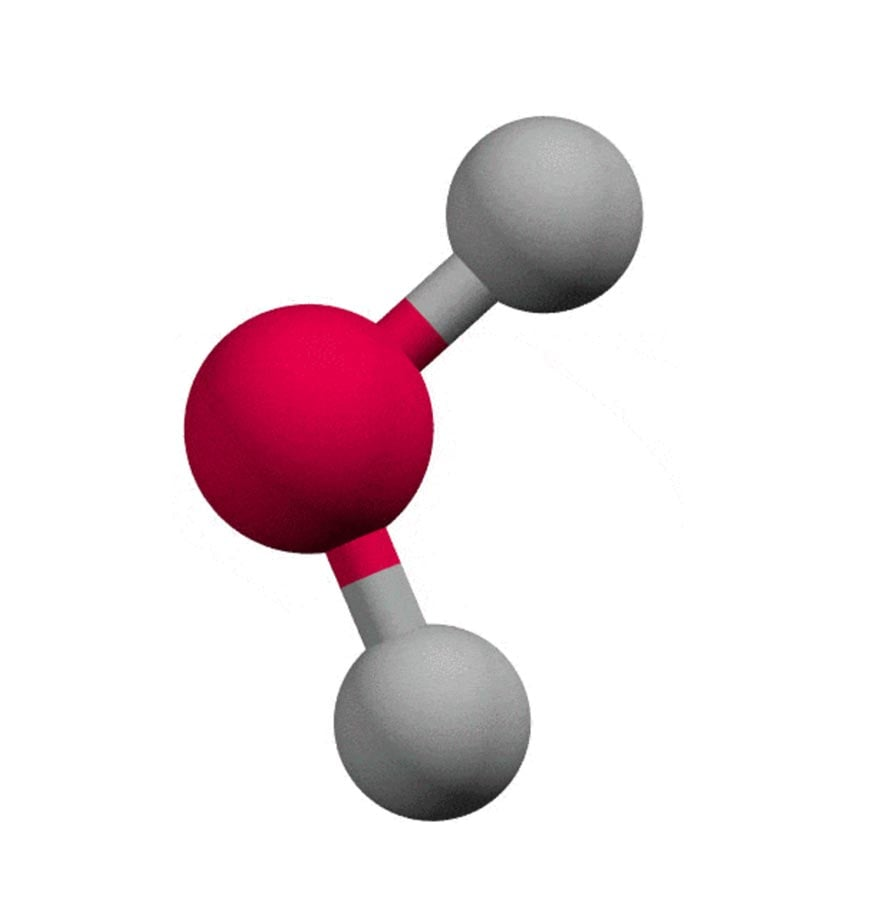Trigonal Planar (Three VSEPR groups)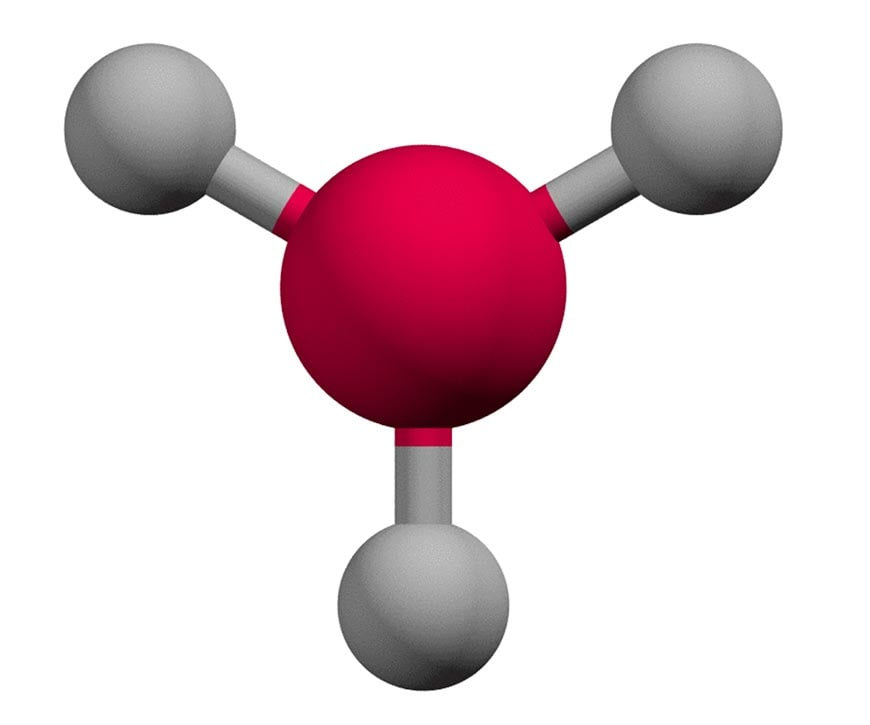Trigonal Pyramid (Four VSEPR groups, one lone pair)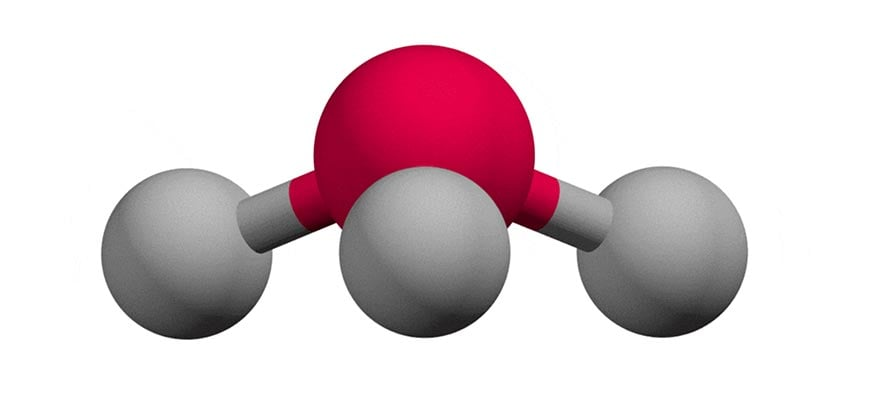Tetrahedral (Four VSEPR groups)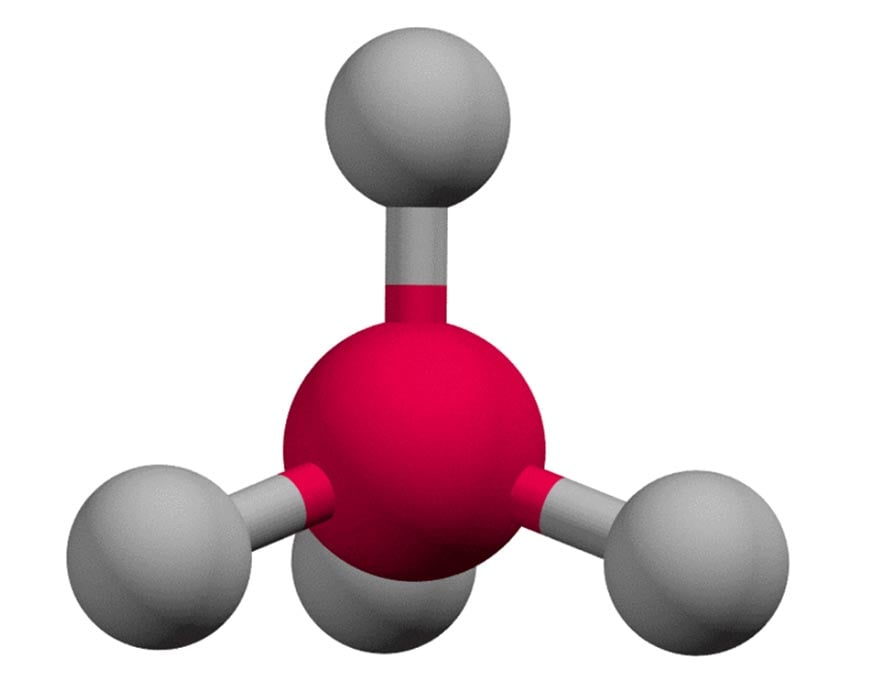### Allotropes

Allotropes are different forms of the same element – each show distinct structural variation, with the atoms of the element bonded together in a different manner.

You should be familiar with different substances that display this property.

For example, diamond and graphite are two allotropes of carbon. Likewise, ozone and dioxygen are two allotropes of oxygen.

### Chemical structure

Elements and compounds are made up of different things, so they have different chemical structures!

The different structures you need to know are illustrated below.

Ionic solids are composed of a crystalline lattice of anions and cations, such as in sodium chloride.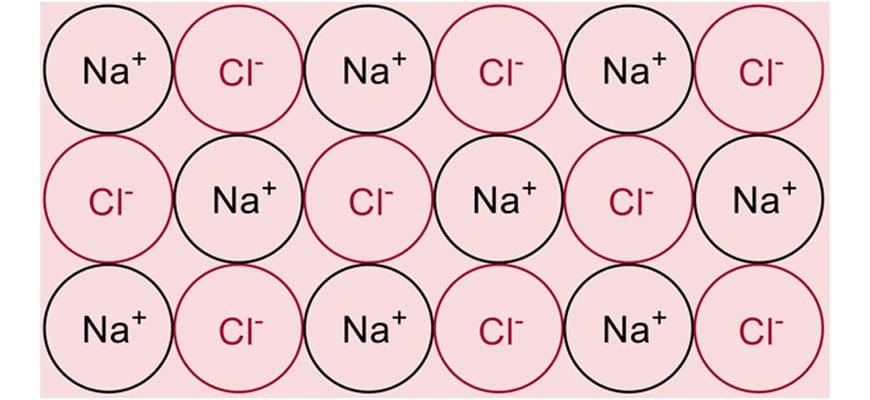In a metallic structure, metal cations are dispersed in a “sea” of delocalised electrons.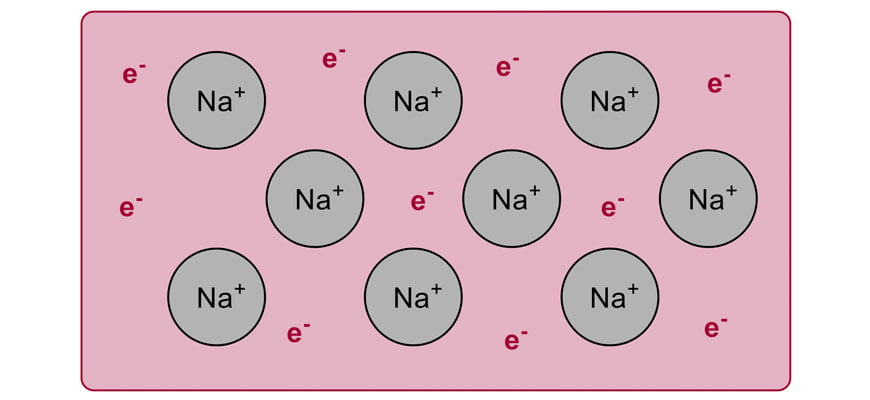Covalent networks are composed of ordered, repeating units joined by covalent bonds.

For example, the diagram below shows one sheet of graphite (composed of many sheets stacked vertically).

In this structure, every carbon atom is bound to three additional carbon atoms, and is highly ordered.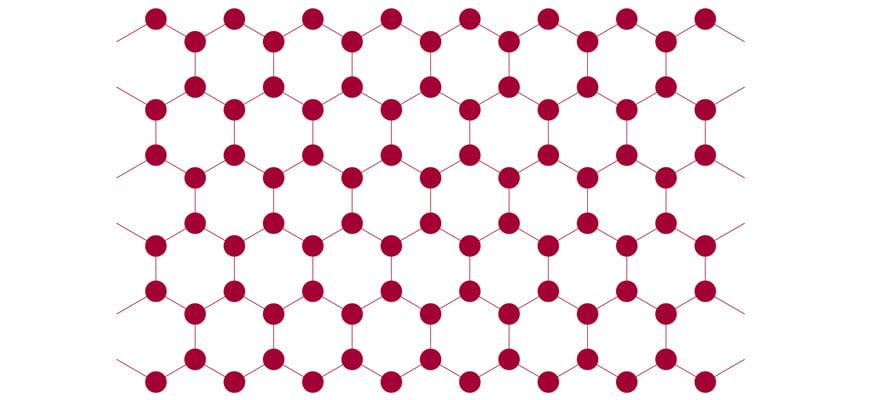Covalent molecular substances contain discrete molecules, and are held together by intermolecular forces.

There are three types of intermolecular forces:

1. Dispersion forces: between all molecules, and increase as molecular mass increases.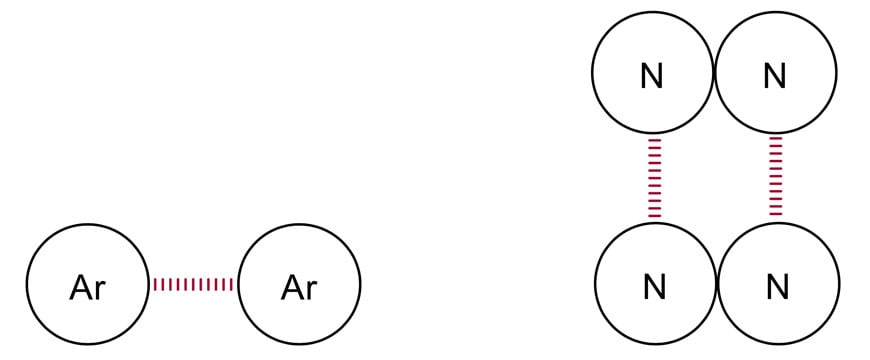2. Dipole-dipole forces: between polar molecules, and increase as the polarity of the molecule increases.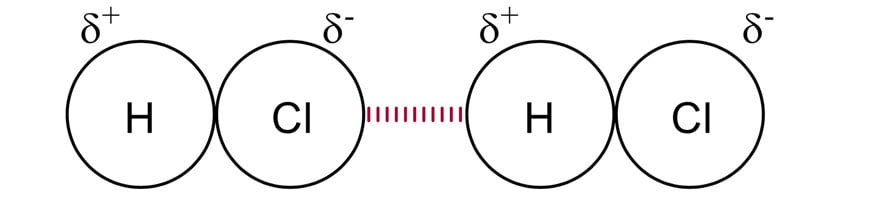3. Hydrogen bonding
: between molecules that have a hydrogen atom directly bound to a nitrogen, oxygen, or fluorine atom.

Hydrogen bonds increase in strength as the number of hydrogen atoms directly bound to nitrogen, oxygen and fluorine increases within a molecule.  Hydrogen bonds also increase in strength from nitrogen to oxygen to fluorine.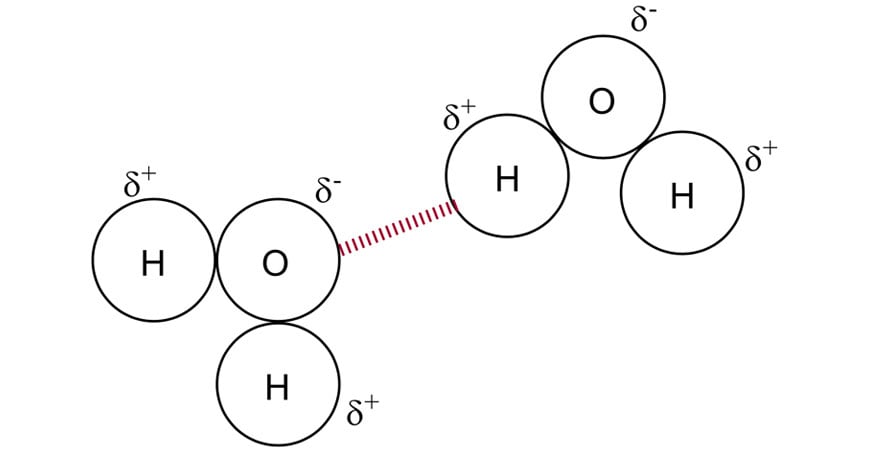Hydrogen bonds are stronger than dipole-dipole forces which are stronger than dispersion forces.

Intermolecular forces are usually weaker than ionic, covalent and metallic bonds.

### The relationship between chemical structure and physical properties

The chemical structure of an element or compound will directly influence the physical properties that are observed for the element or compound.

The following points can help you match the chemical structure of an element or compound to the physical properties of that substance.

• A substance will conduct electricity if charged particles can move within the substance
• A substance will conduct heat if particles are able to move and vibrate within a substance
• A substance will have a high melting point/boiling point if the bonds/forces holding that substance together are strong. Remember, covalent molecular compounds will be held together by intermolecular forces, and covalent bonds are not broken when they melt/boil.
• A substance will be hard if the bonds/forces holding that substance together are strong. A substance will be soft if the bonds/forces holding that substance together are weak.
• Substances are malleable if their chemical structure can be reshaped easily and does not experience repulsion upon deformation.

© Matrix Education and www.matrix.edu.au, 2022. Unauthorised use and/or duplication of this material without express and written permission from this site’s author and/or owner is strictly prohibited. Excerpts and links may be used, provided that full and clear credit is given to Matrix Education and www.matrix.edu.au with appropriate and specific direction to the original content.